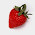# Divisibility Rules: Quantitative Aptitude Section

There are some specific rules by which we can determine the divisor of the given number. Today I will discuss divisibility rules from 2 to 19. Using these rules you can easily determine a divisor of given number, however large it may be. Let me tell you the rules of divisibility from 2 to 19.

## Divisibility Rules

#### Divisibility by 2

Number which ends with even number or 0 is always divisible by 2.
For Example: 44, 120, 56, 70 etc are divisible by 2.
23, 57, 79 etc are not divisible by 3.

#### Divisibility by 3

For a given number, if sum of ts digits is divisible by 3, then the number will be divisible by 3.
For Example: 5676 = 5+6+7+6 = 24, which is divisible by 3.
8912 = 8+9+1+2 = 20, not divisible by 3.

#### Divisibility by 4

Given number will be divisible by 4, if last two digits are divisible by 4 and if number with two or more zeros at end is also divisible by 4.
For Example: 4500, 134000 are divisible by 4.
5228 = last two digits i.e. 28 is divisible by 4, therefore number is divisible by 4.

#### Divisibility by 5

Numbers ends with 5 or 0, will be divisible by 5.
For Example: 465, 670, 565 will be divisible by 5.
556, 877 are not divisible by 5.

#### Divisibility by 6

Number divisible by 3 and 2 both will be divisible by 6. For divisibility by 3, you will use the same rule of 3 as discussed above.
For Example: 154 : Divisible by 2.
1+5+4 = 10 which is not divisible by 3. Therefore, number is not divisible by 6.
3924 : Divisible by 2. Also, 3+9+2+4 = 18, which is divisible by 3. Therefore, number divisible by 6.

#### Divisibility by 7

To check divisibility by numbers like 7, 13, 17 , 19 ,we always use one key digit known as Osculator. Osculators for 7 is -2 i.e. negative osculator 2. Lets see how we will use osculator to check divisibility.

Suppose, we need to check divisibility of number 119,

Last digit of number is to be multiplied with -2 (osculator) and then added to the remaining part of number. Check that whether the resultant number is divisible by 7 or not.
Therefore, number will be divisible by 7, if resultant is divisible by 7.

#### Divisibility by 8

Given number will be divisible by 8, if last three digits are divisible by 8 and number with three  zeros at end is also divisible by 8.
For Example: 4589000, 1256 are divisible by 8.

#### Divisibility by 9

If sum of all digits of a number is divisible by 9, the number will be also divisible by 9.
For Example: 456147 = 4+5+6+1+4+7 = 27 which is divisible by 9. Therefore, number is divisible by 9.

#### Divisibility by 10

Number ends with 0 is always divisible by 10.
For example: 100, 2890, 4560 are divisible by 10.

#### Divisibility by 11

If sums of digits at odd places and even places are equal or differ by a number divisible by 11, then number will be divisible by 11.

For Example: 3245693
Sum of odd digits = 3+4+6+3 = 16
Sum of even digits = 2+5+9 = 16
Sums are equal, therefore give number is divisible by 11.

#### Divisibility by 12

Number divisible by 4 and 3 both will be divisible by 12. For divisibility by 4 and 3, you will use the same rule of 4 and 3 as discussed above.
For Example: 156 : 1+5+6 = 12 which is divisible by 3.
Last two digits 56 are divisible by 4. Therefore, number is divisible by 12.
3924 : Divisible by 4. Also, 3+9+2+4 = 18, which is divisible by 3. Therefore, number divisible by 12.

#### Divisibility by 13

Osculator for 13 = 4. The method is same as 7 as discussed above.
See how we check the divisibility by 13, last digit is multiplied with osculator (4), then added to remaining number.

#### Divisibility by 14

Number divisible by 7 and 2 both will be divisible by 14. To check divisibility by 7 and 2, you will use the same rules as discussed above.

#### Divisibility by 15

Number divisible by 5 and 3 both will be divisible by 15. Divisibility by 5 and 3 will be same as discussed above.

#### Divisibility by 16

Number divisible by 8 and 2 both will be divisible by 16. Divisibility by 8 and 2 will be same as discussed above.

#### Divisibility by 17

Osculator for 17 is -5 (negative osculator). Method is same as discussed in divisibility by 7 and 13.

#### Divisibility by 18

Number divisible by 9 and 2, will be divisible by 18.

#### Divisibility by 19

Osculator for 19 is 2 (negative osculator). Method is similar as discussed in divisibility by 7 and 13.
So, Remember that
Osculator of 7 = -2
Osculator of 13 = +4
Osculator of 17 = -5
Osculator of 19 = +2

#### What's trending in BankExamsToday

Smart Prep Kit for Banking Exams by Ramandeep Singh - Download here1.what is osculator mam? nd how we can find it?

1.Osculators are just key digits you have to use to check divisibility of 7, 13, 17 ,19. I hv discussed the way we use them.
Basically,positive osculator means number needs one more to be multiple of 10.
Negative osculator means number needs one less to be multiple of 10.
Ignore the method how we find it,, just try the method of using them.

2.9 is missing...

Sum of the digits divisible by 9

1.Thnx for informing.. I have updated.

3.If the number is Divisible by more than 3 digits.. is osculator method ll work or not???
If it works?
Please Explain me the method mam

I will try to respond asap